Learn all Concepts of Chapter 4 Class 10 (with VIDEOS). Check - Quadratic Equations - Class 10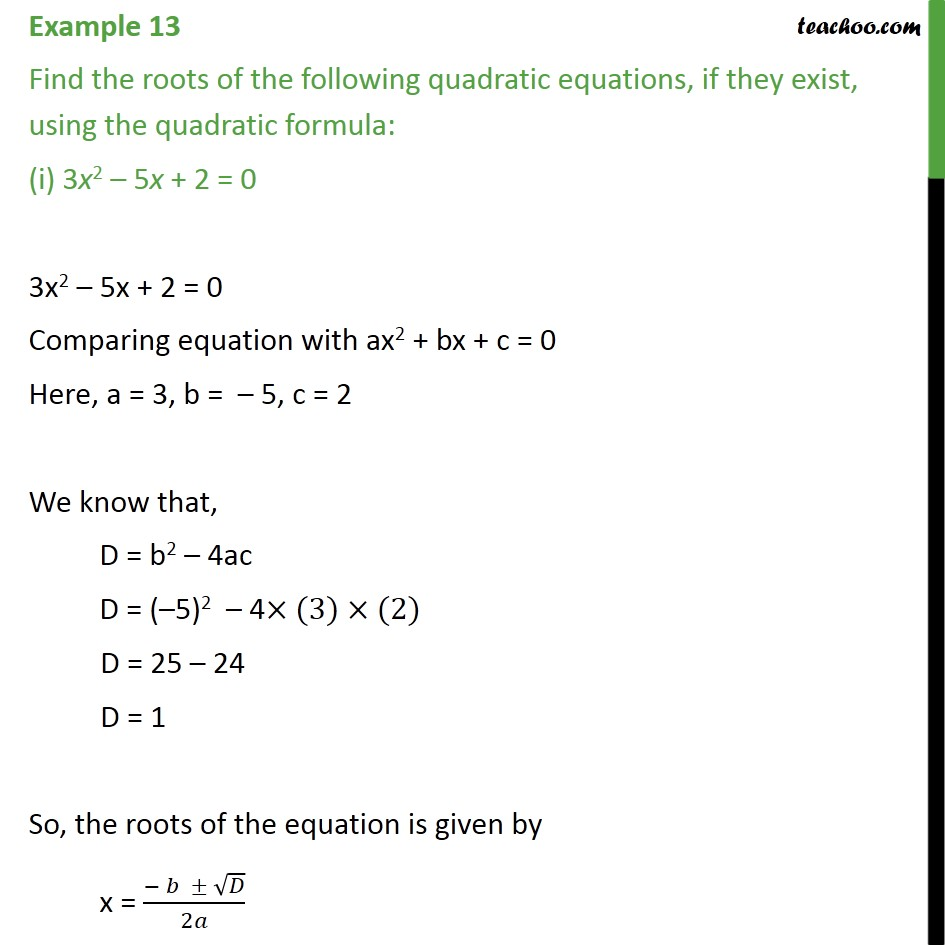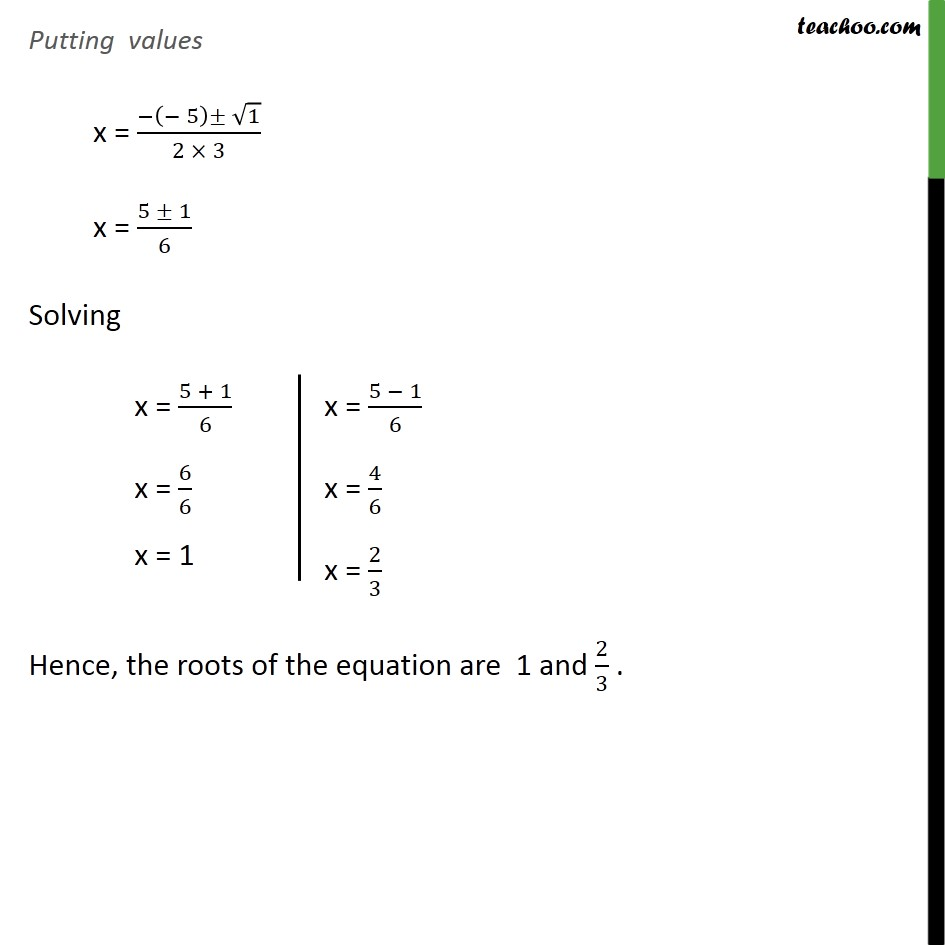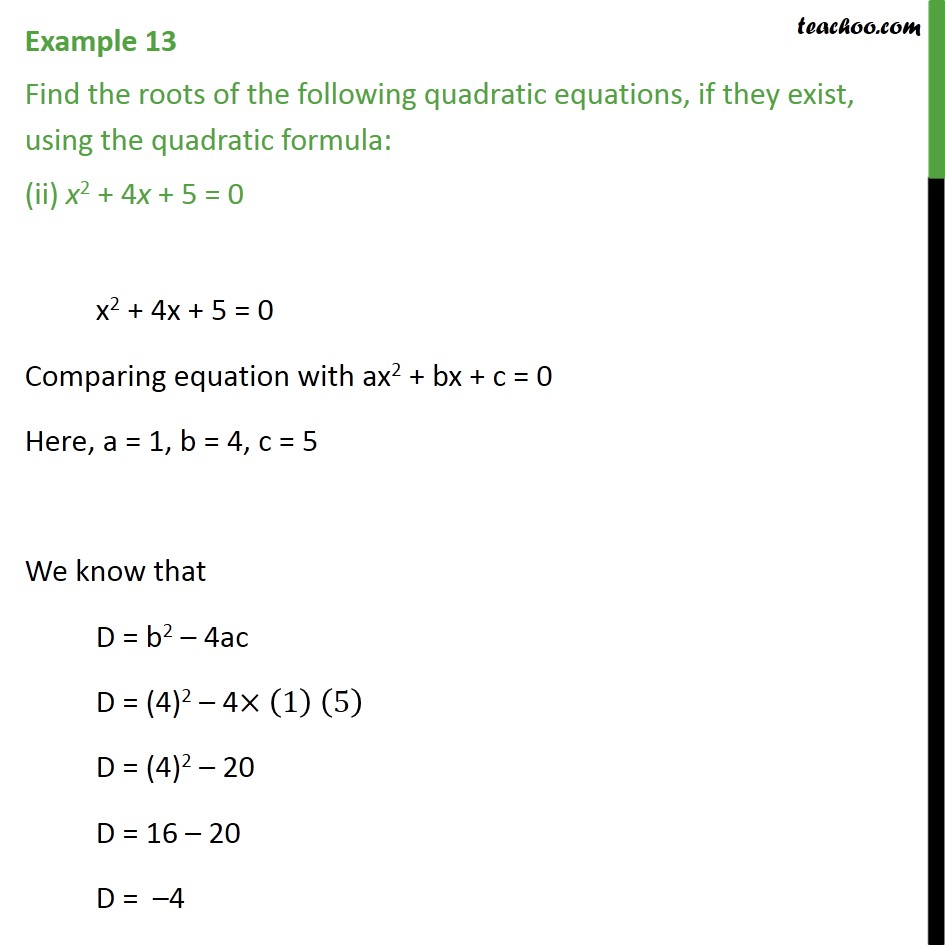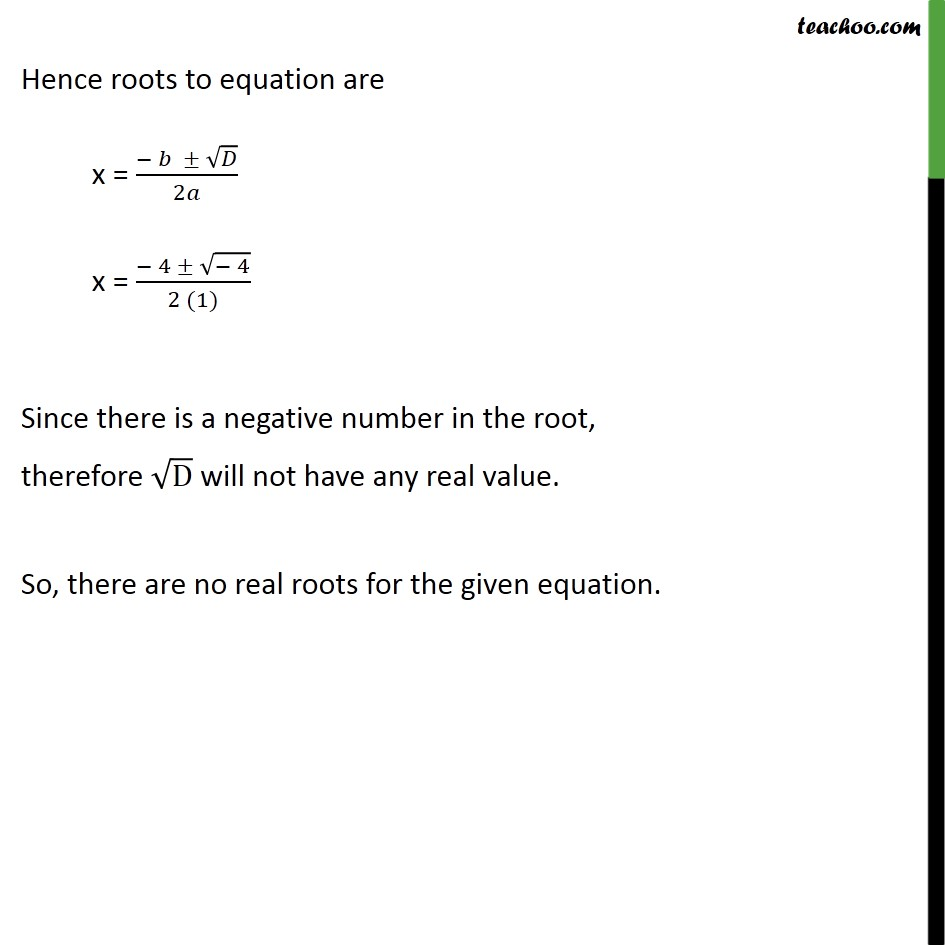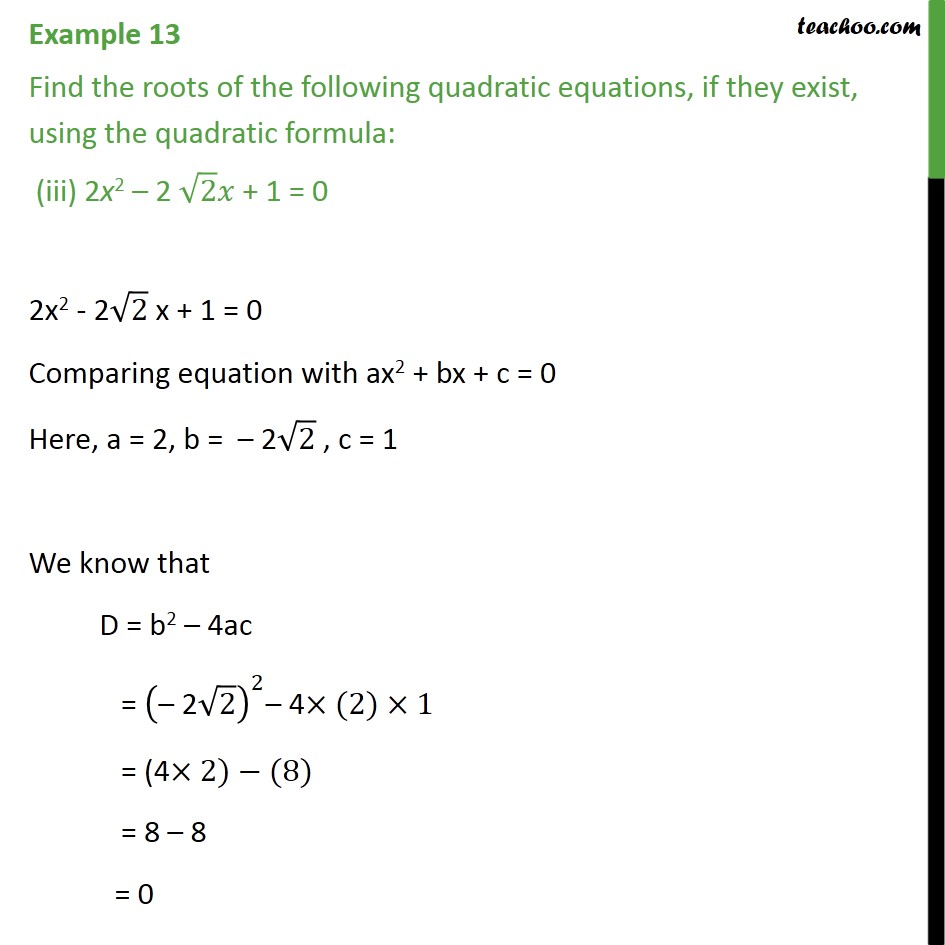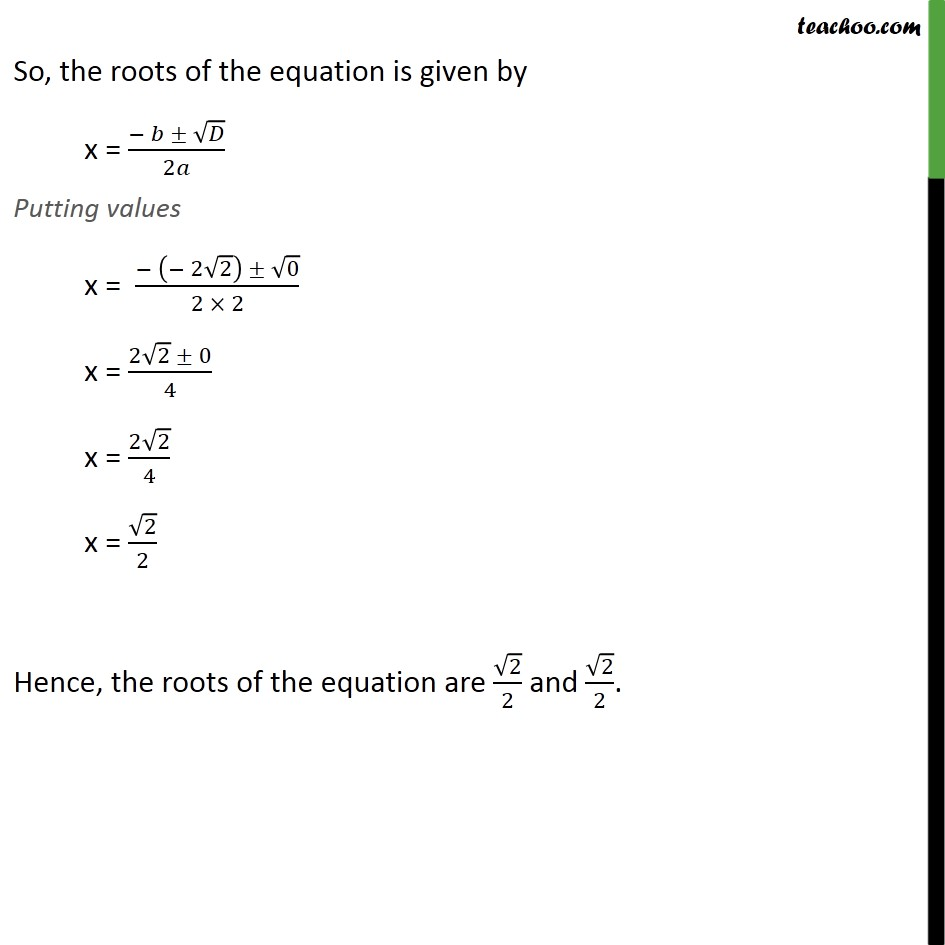1. Chapter 4 Class 10 Quadratic Equations
2. Serial order wise
3. Examples

Transcript

Example 13 Find the roots of the following quadratic equations, if they exist, using the quadratic formula: (i) 3x2 5x + 2 = 0 3x2 5x + 2 = 0 Comparing equation with ax2 + bx + c = 0 Here, a = 3, b = 5, c = 2 We know that, D = b2 4ac D = ( 5)2 4 (3) (2) D = 25 24 D = 1 So, the roots of the equation is given by x = ( )/2 Putting values x = ( ( 5) 1)/(2 3) x = (5 1)/6 Solving Hence, the roots of the equation are 1 and 2/3 . Example 13 Find the roots of the following quadratic equations, if they exist, using the quadratic formula: (ii) x2 + 4x + 5 = 0 x2 + 4x + 5 = 0 Comparing equation with ax2 + bx + c = 0 Here, a = 1, b = 4, c = 5 We know that D = b2 4ac D = (4)2 4 (1) (5) D = (4)2 20 D = 16 20 D = 4 Hence roots to equation are x = ( )/2 x = ( 4 ( 4))/(2 (1)) Since there is a negative number in the root, therefore D will not have any real value. So, there are no real roots for the given equation. Example 13 Find the roots of the following quadratic equations, if they exist, using the quadratic formula: (iii) 2x2 2 2 + 1 = 0 2x2 - 2 2 x + 1 = 0 Comparing equation with ax2 + bx + c = 0 Here, a = 2, b = 2 2 , c = 1 We know that D = b2 4ac = (" 2" 2)^2 4 (2) 1 = (4 2) (8) = 8 8 = 0 So, the roots of the equation is given by x = ( )/2 Putting values x = ( ( 2 2) 0)/(2 2) x = (2 2 0)/4 x = (2 2)/4 x = 2/2 Hence, the roots of the equation are 2/2 and 2/2.

Examples